﻿ A Graph-based Technique for the Spectral-spatial Hyperspectral Images Classification

### A Graph-based Technique for the Spectral-spatial Hyperspectral Images Classification

F. Poorahangaryan, H. Beheshti, S.A. Edalatpanah

## A Graph-based Technique for the Spectral-spatial Hyperspectral Images Classification

F. Poorahangaryan1, H. Beheshti1, S.A. Edalatpanah1,1Department of Electrical and Computer Engineering, Ayandegan Institute of Higher Education, Tonekabon, Iran

### Abstract

Minimum Spanning Forest (MSF) is a graph-based technique used for segmenting and classification of images. In this article, a new method based on MSF is introduced that can be used to supervised classification of hyperspectral images. For a given hyperspectral image, a pixel-based classification, such as Support Vector Machine (SVM) or Maximum Likelihood (ML) is performed. On the other hand, dimensionality reduction is carried out by Principal Components Analysis (PCA) and the first eight components are considered as the reference data. The most reliable pixels, which are obtained from the result of pixel-based classifiers, are used as markers in the construction of MSF. In the next stage, three MSF’s are created after considering three distinct criteria of similarity (dissimilarity). Ultimately, using the majority voting rule, the obtained classification maps are combined and the final classification map is formed. The simulation results presented on an AVRIS image of the vegetation area indicate that the proposed technique enhanced classification accuracy and provides an accurate classification map.

• F. Poorahangaryan, H. Beheshti, S.A. Edalatpanah. A Graph-based Technique for the Spectral-spatial Hyperspectral Images Classification. International Transaction of Electrical and Computer Engineers System. Vol. 4, No. 1, 2017, pp 1-7. http://pubs.sciepub.com/iteces/4/1/1
• Poorahangaryan, F., H. Beheshti, and S.A. Edalatpanah. "A Graph-based Technique for the Spectral-spatial Hyperspectral Images Classification." International Transaction of Electrical and Computer Engineers System 4.1 (2017): 1-7.
• Poorahangaryan, F. , Beheshti, H. , & Edalatpanah, S. (2017). A Graph-based Technique for the Spectral-spatial Hyperspectral Images Classification. International Transaction of Electrical and Computer Engineers System, 4(1), 1-7.
• Poorahangaryan, F., H. Beheshti, and S.A. Edalatpanah. "A Graph-based Technique for the Spectral-spatial Hyperspectral Images Classification." International Transaction of Electrical and Computer Engineers System 4, no. 1 (2017): 1-7.

 Import into BibTeX Import into EndNote Import into RefMan Import into RefWorks

1
Prev Next

### 1. Introduction

In hyperspectral images, each pixel contains information of more than one hundred spectral bands regarding the physical nature of the different materials. Hyperspectral image classification, or simply assigning a class to each image pixel, has various applications. Due to the occurrence of Hughes Phenomenon, however, classification accuracy usually faces difficulties. Hughes Phenomenon occurs when a large number of the features (spectral bands) coupled with limited of training. In order to overcome this phenomenon, the reduction of spectral bands is inevitable. Common dimension reduction algorithms such as PCA , ICA , LDA , KPCA , e.g. are introduced in this regard.

Several techniques have been proposed for the purposes of spectral image classification. Methods like Maximum Likelihood (ML) , Decision Tree , and kernel-based techniques such as Support Vector Machine (SVM) [7, 8, 9] use spectral data of images to classification. With the advancements in imaging technology equipment, the spatial resolution of the images has increased. Researchers have therefore recognized the advantages of using spatial information in the process of classification. Spatial information refers to the information obtained from neighbouring pixel. Previous research indicates that those techniques that use spatial-spectral information in classification are more effective, suitable and robust than those techniques that only use spectral information . Various methods have been proposed for the implementation of spatial information. For example, morphological profiles [10, 11], Markov Random Fields (MRF) [12, 13] have widely been used to determine spatial structures.

A powerful tool for determining spatial structure and including spatial information in the process of classification is image segmentation. In image segmentation, an image is divided into homogenous regions based on the criteria of homogeneity. The criteria of homogeneity can be intensity or texture. In a segmentation map, each region is a spatial neighbourhood for all the pixels of this region. Thus, an adaptive adjacency is formed when it is more efficient than when local adjacency-based techniques, such as techniques based on fixed window. The algorithms of Watershed  and partition clustering  have widely been used in this field. Tarabalka et al.  introduced an minimum spanning forest (MSF) for segmentation and classification of hyperspectral images. With this technique, the selection of markers for region growth is done automatically and, as a result, over-segmentation and under-segmentation will decline. In their proposed algorithm, SVM classification results are used to create the probability map and to discover more reliable pixels as the markers. It is also possible to use the results of multiple spatial-spectral classifications to find the markers . Then, image pixels are grouped into an MSF, where each tree is rooted on a obtained marker. MSF belongs to graph-based methods of image segmentation. In these methods, an image is replaced with a graph in a way that each pixel is considered as a graph vertex, and graph edges connect the vertices. Every edge is assigned with a weight, which shows similarity (or dissimilarity) between two pixels (vertices). In 2011 , a group of researchers used random markers to create MSF. In this method, the M marker map, and thus, M MSF are constructed. Then, using the majority vote rule the final spatial-spectral classification map is produced. A significant advantage of this method can be the absence of any challenges as to how select the marker.

In the mentioned methods, two common similarity (dissimilarity) criteria such as L1 vector norm and Spectral Angel Mapper (SAM) were used to assign weights to graph edges L1 vector norm calculates the spectral distance between two vectors. The SAM distance computes the angel between two pixel vectors for determining the spectral similarity them. An examination of the previous studies obviously shows that in some cases L1 led to better results. However, it was SAM that delivered better results in most cases. Therefore, it can be inferred that the outcome of using each of these criteria depends on the nature of the image. In case an algorithm was able to take advantage of both criteria, it would be possible to achieve better results. Furthermore in this study, since D1 similarity criterion has demonstrated its efficiency in other fields , effect of the combination of this measure with other common criteria such as L1 and SAM is dealt with. On other hand, this study focuses on expanding upon this technique to increase classification accuracy by combining the results of various similarity (dissimilarity) measures that are associated to each edge of the graph.

This paper is divided into the following sections: the next section provides us with a classification structure based on MSF. The experimental results are presented and discussed in Section 2 and Section 3 deals with the final conclusion of the study.

### 2. Proposed Spectral-Spatial Classification FrameworkDownload asVeiw figureFigures index
Figure 1. Scheme of proposed MSF based spectral-spatial classification

The flowchart of the proposed classification technique is presented in Figure 1. The input is a hyperspectral B-band and the output is an image in which each pixel is assigned to one of the considered n classes. The introduced algorithm is composed of four main steps as follows:

1) pixelwise classification; The aim of this step is to first provide a supervised classification map from a hyperspectral image. Marker selection is performed base on the results of this step.

2) Marker Map Production; the aim of this step is to choose the most reliable classified pixels in order to define suitable markers. The obtained markers are used on MSF construction

3) Dimension Reduction; High-dimensionality data processing also requires huge computational resources and storage capacity. Meanwhile, the spectral bands are often correlated, and not all of them are useful for the specific classification task. Therefore, a dimension reduction procedure is required.

4) MSF Construction; the aim of this step is the spatial–spectral classification using marker-controlled MSF;

5) Majority voting: the aim of this step is obtaining of final classification map. In this step, the majority vote rule is used for class assignment to each pixel

Each stage of the flowchart will be described in the following paragraphs.

2.1. Pixel-wise Classification

In the first stage of the flowchart, pixel-based classifications are applied. Two pixel-based classifiers are proposed that demonstrate desirable performances in the classification of HSIs: Support Vector Machine (SVM), and Maximum Likelihood (ML). The results obtained from these classifiers are utilized in the production of the marker map.

2.1.1. Support Vector Machine (SVM) Classifier

SVM has been the classifier of choice for many researchers in solving different problems of classification in real world. In this section, a general description of SVM is provided. A supervised classification problem is typically stated in this way: Using n training samples of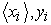where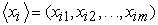is the input feature vector andis the label of the classification class, the discriminant function or classifier learns the existing classification of the models in the learning samples in a way that it can allocate an unknown but appropriate xi or yi input. SVM was essentially designed for such conditions; i.e. binary classification. However, it requires the capacity of development in multiclass conditions. Like other linear classifications, SVM attempts to form a linear decision boundary (supposing data are linearly discriminable) or a linear hyperplane between two classes.

It is generally believed that the points of two distinct classes are positioned in a way that there appears a distance between them. SVM attempts to maximize such a distance after considering the problem as a second-order planning problem. In a non-linear condition, a mapping function such as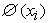is used to map the input space into a feature space in higher dimensions as to the non-linear hyperplane turns into a linear one. In order to prevent the increase in calculation complexities and the problems with working in higher dimensions, a K (xi,xj) core is utilized, and it essentially calculates the core equal to the input space so that there would be no need for explicit mappings .

As was mentioned, normal SVM is only capable to separate the two classes. There are two main strategies to classify more than two classes  :

- One against One (OAO): This strategy separates each pair of classes. Thus, for a classification problem with n classes, SVM is applied n(n − 1)/2 times to distinguish samples of one class from samples of another class. The classification result of an unlabeled sample is based on the majority voting, where each SVM votes for one class.

- One against all (OAA): In this strategy, there is a classifier for each pixel. So if the number of classes is n, SVM used n times. In order to train nth classifier, the samples of nth class are used against other training samples.

2.1.2. Maximum Likelihood (ML) Classifier

This classifier belongs to the class of supervised classifications based on the Bayesian theory in which the frequency function of the classes is presumed normal. Thus, in samples with B spectral band with the class frequency function is presented as follows.(1)

where, P(x|ci) is the ith class posterior probability, µi and Ci are respectively the mean vector and ith class covariance matrix, which are calculated with the learning data of each class. Using natural logarithm and after eliminating shared factors among classes, the discriminant function is calculated according to the following relation.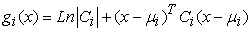(2)

Using this relation, that class for which the discriminant function is the minimum is selected as the sample class .

2.2. Marker Map Production

The markers would be selected from the results obtained from two previous pixel-based classifiers. If a pixel is assigned to a single class by both classifiers, that pixel is considered as the marker. The label for these markers is the same as the label picked up from the results of the initial pixel-wised classification. Other pixels are assigned with “0” label. Figure 2 shows the formation of the marker map. Among others, the simplicity of this method is a noticeable benefit. However, it has a noticeable shortcoming, too: when the marker is mistakenly assigned to a class, the tree grown from that marker would yield incorrect labels.Download asVeiw figureFigures index
Figure 2. Formation of a marker map from result of pixel-wised classifiers
2.3. Dimension Reduction

The classification resolution of HSIs has always been challenging because of the large dimensions of such images. As a result, reducing the dimensions (reducing quality bands) in HSIs is a necessary requirement. The most common reduction algorithm is Principal Components Analysis (PCA). PCA finds an orthogonal projection to maximize the global data variance. For more details, see Ref. .

2.4. MSF Construction

The results of the previous two stages are used to create the MSF. For this purpose, each pixel is considered as a vertex v of an undirected graph G = (V,E,W), where V and E are the set of vertices and edge, respectively, and W is a mapping of the set of edge E into R2. Each edge eij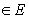links a couple of vertices i and j corresponding to the neighbouring pixel. Furthermore, a weight wij is assigned to each edge eij, which the degree of dissimilarity between two pixels connected by this edge. The proposed dissimilarity measures in previous works are consisting of a L1vector norm, spectral angle mapper (SAM) and spectral information diverges (SID). L1 vector norm and SAM between two pixel vectors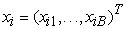andare obtained as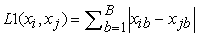(3)(4)

where, B is the number of spectral bands and xib is the spectral amount of ith pixel in bth spectral bands.

In this study, L1 and SAM soft vectors were evaluated. Besides, another criterion called D1 vector is introduced. This norm vector have been proved applicable in other areas . In this study, therefore, the application of this criterion for segmenting hyperspectral images is recommended. The D1 vector norm ensure compares the difference in spectral shape instead of intensity as the L1 vectors norm class. The D1 vector norm first converts the spectral information for each pixel from an intensity measurement to a measurement of the spectral shape. This resulting vector can then be compared using an L1 vector norm comparison and is given by:(5)

After achieving similarity vector, the next stage is to create the improved vector for the purpose of allocating a weight to each edge. The improved graph is reached by adding added ri vertexes to the main graph in accordance with m markers. Each added ri vertices is linked with an edge with a weight of 0 to the marker i. The minimum spanning tree of the constructed graph induces an MSF in G, where each tree is grown on a vertex ri; the MSF is obtained after removing the vertex ri. Prim’s algorithm  is employed to create MSF. This algorithm has the following structure.

- Start: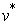is defined as a set of extra vertices

-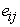with minimal weight of the modified graph is selected such that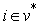and-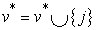-If, return to start .

In this paper, L1, SAM, and D1 similarity criteria were used, and three MSF-based classification maps were constructed. The next stage would be the combination of the achieved classification maps to take advantage of all three criteria.

2.5. Majority Voting

In this stage of the algorithm, there are three spatial-spectral classification maps with regard to the three criteria of similarity measurement (dissimilarity measurement) between two pixels when creating MSF. In other words, for each pixel exists a 1*3 vector. According to the rule of majority, each pixel is assigned with a class which has been repeated more than others. Finally, the ultimate classification map is formed. By effective combination of classification results, the use of information contained in each of them is provided.

### 3. Results and Discussion

The Indian Pines image is taken from a vegetation area, which is registered by AVRIS sensor. This image is 145 by 145 pixels, with a spatial resolution of 20 m/pixels. After eliminating twenty water absorption bands, a 200-band image was used for the purpose of experiments. The reference image of this data can be seen in Figure 3.(a). The 200-band image will be used to pixel-wised classification. Moreover, after the application of PCA, eight principal components will be utilized in the construction of MSF. We have randomly chosen 50 training samples in each class – from classes “alfalfa”, “grass/pasture-mowed” and “oats” to perform pixel-wised ML and SVM classifications. Since the three mentioned classes have few samples in the reference map, 15 samples were selected of them. Multiclass SVM was utilized in One-Against-one (OAO) mode with RBF function to simulate MATLAB.

The efficiency of the method is evaluated in terms of the following two qualities:

1) Mathematical efficiency: classification accuracy measures [class-specific accuracy (CA) and average accuracy (AA)];

2) Visual accuracy: visual comparison between the obtained classification maps and reference map.

Assuming the following definitions:

- Class Accuracy: the CA is the percentage of correctly classified pixel for each class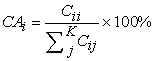(6)

- Average Accuracy: The AA is average of CA values in all of classes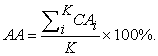(7)

The classification accuracy of the two pixel-wised ML and SVM classifiers and the proposed technique are provided in Table 1. This table indicates that the accuracy of classification has improved after the combination of spatial and spectral maps on the basis of MSF for various criteria. This outcome is consistent with the achieved classification maps. The SVM (Figure 3.(b)) and ML (Figure 3.(c)) classification map has high amounts of noise, but the obtained classification map from our framework (Figure 3.(d)) was closer to the reference.Download asVeiw figureFigures index
Figure 3. Indiana image, (a) Reference data. (b) Pixel-wised classification map, using SVM. (c) Pixel-wised classification map, using ML. (d) Classification map obtained by the proposed scheme

#### Table 1. INFORMATION CLASSES AND COMPARISONS OF ACCURACIES OF THE THREE METHODSDownload asPowerPoint Slide

Veiw figure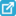View current table in a new window

Table 1 clearly demonstrates that the proposed classification yields higher accuracy than pixel-wised classifiers. The proposed method yields the best accuracy, improving the AA by 10.65 and 24.06 percentage points, when compared to the SVM and ML classification, respectively. It is easy to see that our model is better than SVM, except in the classes “Hay-windrowed” and “Wheat”. The spectral within-class variability exists across the study site. This variability stems from the diversity of materials in the observed area, object geometry, illumination effects, atmospheric interaction, and spectral resolution of the sensors as well as spectral mixing effects as a function of the spatial sensors resolution. Indian Pines is a dataset with low spatial resolution. Low spatial resolution leads to the existence of mixed pixels. So, within-class variability increases in low spatial resolution images. Moreover in Indian scene, the measured spectrums for a plant in different areas are very different from each other. Therefore, the probability distribution of classes is multi-modal. The spectrum values of samples in some available classes e.g. “Hay-windrowed” and “Wheat” are close together. It means that they have low within-class variability. Therefore, there are a few outlier samples in the mentioned classes and based on these situations the SVM works very well.

### 4. Conclusions

Although the increase in the number of spectral bands in hyperspectral images enhances the potential for object identification and class discrimination, the classification of such images faces difficulties due to the occurrence of Hughes Phenomenon. Thus, dimension reduction would be inescapable. In addition, the application of spatial information can be the cause of accuracy improvements in classification processes. In this paper, a new spatial-spectral classification for hyperspectral images classification is introduced. This framework rests on the formation of MSF on the basis of three distinct criteria for measuring similarity (dissimilarity) of two pixels and the combination of their results. The MSF will be constructed on the markers obtained from multiple pixel-based classifiers. Various criteria have been proposed for the measurement of the similarity of two pixels in MSF construction. The results of the experiments on Indian pines data indicate that although D1 criterion yielded better results than SAM and L1, the rule of majority shows that the combination of the classification result of these three criteria has enhanced classification accuracy. The proposed algorithm has the following advantages:

1. Due to the existence of spatial information in the process of classification, the classification accuracy has increased compared with pixel-wised classifications.

2. Due to the combination of the particular features of each of the three criteria of pixel similarity measurement during the formation of MSF, the classification resolution has improved over the times when each criterion is implemented separately.

### References

  L. J. P. Maaten, E. O. Postma, and H. J. Herik, “Dimensionality reduction:A comparative review,” Univ. Maastricht, Amsterdam, The Netherlands, Tech. Rep, 2009.In article PubMed  S. Moussaoui, H. Hauksdottir, F. Schmidt, C. Jutten, J. Chanussot,D. Brie, S. Douté, and J. A. Benediktsson, “On the decomposition of Mars hyperspectral data by ICA and Bayesian positive sourceseparation,” Neurocomputing, vol. 71, no. 10-12, pp. 2194-2208, 2008.In article View Article  R. Dianat and Sh. Kasaei, “Dimension Reduction of Optical RemoteSensing Images via Minimum Change Rate Deviation Method,” IEEE Trans.Geosci. Remote Sens., Vol. 48, no. 1, pp. 198-206, 2010.In article View Article  H. Huang, J. Liu and Y. Pan, “Semi-Supervised Marginal Fisher Analysisfor Hyperspectral Image Classification,” XXII ISPRS Congress, pp.377-382, 2012.In article  D. A. Landgrebe, Signal Theory Methods in Multispectral Remote Sensing. NewYork: Wiley, 2003.In article View Article  PubMed  H. Zhou, Z. Mao, and D. Wang, “Classification of coastal areas by airborne hyperspectral image,” in Proc. SPIE Opt. Technol. Atmos., Ocean,Environ. Stud., vol. 5832, pp. 471-476, 2005.In article  C.H. Li, B.C. Kuo, C.T.Lin and C. S. Huang, “A Spatial–Contextual Support Vector Machinefor Remotely Sensed Image Classification,” IEEE Trans. Geosci. Remote Sens., vol. 50, no. 3, pp. 784-799, 2012.In article View Article  M. Fauvel, J. Chanussot, and J. A. Benediktsson, “Evaluation of kernels for multiclass classification of hyperspectral remote sensing data,” in Proc. ICASSP, pp. II-813-II-816, 2006.In article View Article  Mountrakis, G., Im, J., & Ogole, C., “Support vector machines in remote sensing: A review.” ISPRS Journal of Photogrammetry and Remote Sensing, vol.66, no. 3, pp. 247-259, 2011.In article View Article  F. Mirzapour and H. Ghassemian, “Improving hyperspectral image classification by combining spectral, texture, and shape featuresImproving hyperspectral image classification by combining spectral, texture, and shape features”, International Journal of Remote Sensing, vol 36, no. 4, pp. 1070-1096, Feb. 2015.In article  M. Dalla Mura, J.A. Benediktsson B. Waske and L. Bruzzone, “Extended profiles with morphological attribute filters for the analysis classification of hyperspectral data ,” Int. J. Remote Sens., vol. 31, no. 22, pp. 5975-5991,2010.In article View Article  J.. Li, J. M. Bioucas-Dias, and A. Plaza, “Spectral-spatial hyperspectral image segmentation using subspace multinomial logistic regression and Markov random fields,” IEEE Trans. Geos. Remote Sens., vol. 50, no. 3, pp. 809-823, 2012.In article View Article  M. Borhani and H. Ghassemian, “Hyperspectral Image Classification Based on Spectral-Spatial Features Using Probabilistic SVM and Locally Weighted Markov Random Fields ”, Iranian Conference on Intelligent Systems (ICIS 2014), pp. 1-6, 2014.In article View Article  Y. Tarabalka, J, Chanussot, and A. Benediktsson, “ Segmentation and classification of hyperspectral images using watershed transformation,” J. Pattern Recognition, vol. 43, no. 7, pp. 2367-2379, 2010.In article View Article  Y. Tarabalka, J. A. Benediktsson, and J, Chanussot, “Spcetral-spatial classification of hyperspectral imagery based on partitional clustering techniques,” IEEE Trans. Geo. and Remote Seng, vol. 47, no. 8, pp, 2373-2987, 2009.In article PubMed  Y. Tarabalka, J. Chanussot, and J.A. Benediktsson, “Segmentation and classification of hyperspectral images using minimum spanning forest grown from automatically selected markers,” IEEE Trans. Systems, Man, and Cybernetics: Part B, vol. 40, no. 5, pp. 1267-1279, Oct. 2010.In article  Y. Tarabalka, J.A. Benediktsson, J. Chanussot, and J.C. Tilton, “Multiple spectral-spatial classification approach for hyperspectral data,” IEEE Trans. Geosci. Remote Sens, vol. 48, no. 11, pp. 4122-4132, Nov. 2010.In article View Article  K. Bernard, Y. Tarabalka, J. Angulo, J. Chanussot and J. A. Benediktsson, “Spectral–Spatial Classification of Hyperspectral Data Based on a Stochastic Minimum Spanning Forest Approach,” IEEE Trans. Geosci. Remote Sens, vol. 21, NO. 4, pp. 2008-2021, APRIL 2012.In article PubMed  R. Pike, S. K. Patton, G. Lu, L. V. Halig, D. Wang, Z. G. Chen, and B. Fei, “A Minimum Spanning Forest Based Hyperspectral Image Classification Method for Cancerous Tissue Detection”, Conference: Proc. SPIE 9034, Medical Imaging 2014.In article  Cortes, C.; Vapnik, V. “Support-vector networks”. Machine Learning, vol 20, no. 3. pp 273-297, 1995.In article View Article  R.O. Duda, P.e. Hart, and D.G. Stock, “Pattern classification”, Second Edition, wiely, 2001.In article  T. H. Cormen, C. E. Leiserson, R. L. Rivest, and C. Stein, Introduction to Algorithms, 2nd ed. Cambridge, MA: MIT Press, 2001.In article